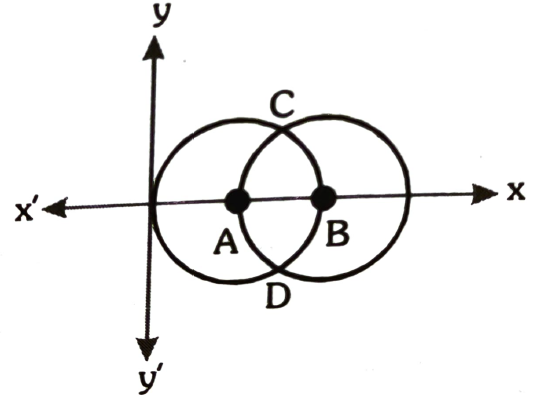Geometry of complex numbers
Question

# If the complex number z is such that $|\mathrm{z}-25|\le 25$ and $|\mathrm{z}-50|=25$ then the minimum value of $|\mathrm{z}|+25$ is 10k  then k is equal to

Moderate
Solution

## $|\mathrm{z}-25|\le 25$ represent interior and boundary of circle withcentre (25, 0) and radius 25. Points z satisfying  $|\mathrm{z}-25|\le 25$and  $|\mathrm{z}-50|=25$  lie on arc DAC hence min $\left\{|\mathrm{z}|\right\}=\mathrm{OA}=25$

Get Instant Solutions